## Example Questions

### Example Question #1 : How To Find Negative Cosine

If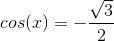and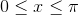, what is the value of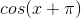?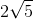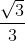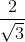Explanation:

Based on this data, we can make a little triangle that looks like: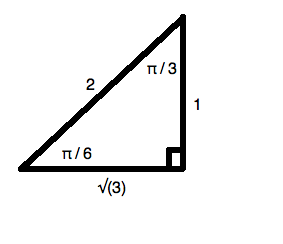This is because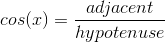.

Now, this means thatmust equal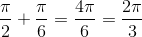.  (Recall that the cosine function is negative in the second quadrant.) Now, we are looking for: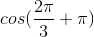or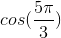.  This is the cosine of a reference angle of: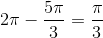Looking at our little triangle above, we can see that the cosine of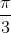is.

### Example Question #2 : How To Find Negative Cosine

What is the cosine of the angle formed between the origin and the point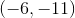if that angle is formed with one side of the angle beginning on the-axis and then rotating counter-clockwise to? Round to the nearest hundredth.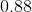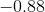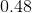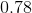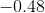Explanation:

Recall that when you calculate a trigonometric function for an obtuse angle like this, you always use the-axis as your reference point for your angle. (Hence, it is called the "reference angle.")

Now, it is easiest to think of this like you are drawing a little triangle in the third quadrant of the Cartesian plane. It would look like: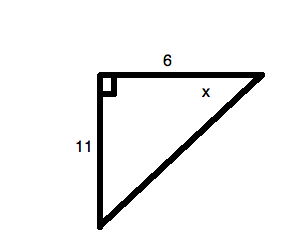So, you first need to  calculate the hypotenuse. You can do this by using the Pythagorean Theorem,, whereandare the lengths of the legs of the triangle andthe length of the hypotenuse. Rearranging the equation to solve for, you get: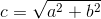Substituting in the given values: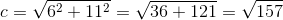So, the cosine of an angle is: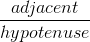or, for your data,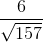.

This is approximately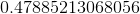. Rounding, this is. However, sinceis in the third quadrant your value must be negative:.

### Example Question #3 : How To Find Negative Cosine

What is the cosine of the angle formed between the origin and the point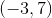if that angle is formed with one side of the angle beginning on the-axis and then rotating counter-clockwise to? Round to the nearest hundredth.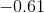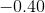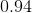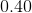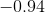Explanation:

Recall that when you calculate a trigonometric function for an obtuse angle like this, you always use the-axis as your reference point for your angle. (Hence, it is called the "reference angle.") Now, it is easiest to think of this like you are drawing a little triangle in the second quadrant of the Cartesian plane. It would look like: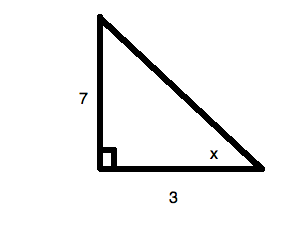So, you first need to  calculate the hypotenuse: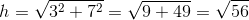So, the cosine of an angle is:or, for your data,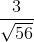.

This is approximately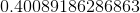. Rounding, this is. However, sinceis in the second quadrant your value must be negative:.

### Example Question #4 : How To Find Negative Cosine

To the nearest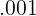, what is the cosine of the angle formed between the origin and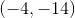? Assume a counterclockwise rotation.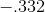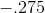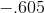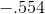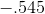Explanation:

If the point to be reached is, then we may envision a right triangle with sidesand, and hypotenuse. The Pythagorean Theorem tells us that, so we plug in and find that: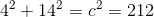Thus,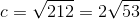Now, SOHCAHTOA tells us that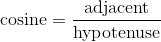, so we know that: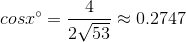Thus, our cosine is approximately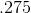. However, as we are in the third quadrant, cosine must be negative! Therefore, our true cosine is.

### Example Question #5 : How To Find Negative Cosine

On a grid, what is the cosine of the angle formed between a line from the origin toand the x-axis?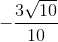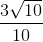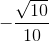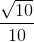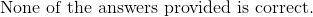Explanation:

If the point to be reached is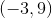, then we may envision a right triangle with sidesand, and hypotenuse. The Pythagorean Theorem tells us that, so we plug in and find that: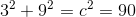.

Thus,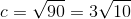.

Now, SOHCAHTOA tells us that, so we know that: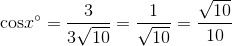Thus, our cosine is approximately. However, as we are in the second quadrant, cosine must be negative! Therefore, our true cosine is.